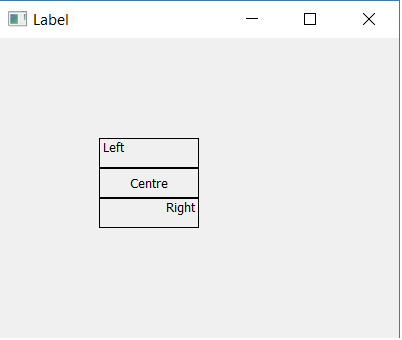Open in App
Not now

# PyQt5 – How to align Text of Label

• Last Updated : 12 Jul, 2022

In this article, we will see how we can align text of labels in PyQt5 application, we can align text in three different ways which are left, right and Center.
Syntax :

```label.setAlignment(QtCore.Qt.AlignLeft)
label.setAlignment(QtCore.Qt.AlignCenter)
label.setAlignment(QtCore.Qt.AlignRight)```

In order to use this we have to import Qtcore from PyQt5

`from PyQt5 import QtCore`

Below is the implementation :

## Python3

 `# importing the required libraries` `from` `PyQt5.QtWidgets ``import` `*``from` `PyQt5 ``import` `QtCore``from` `PyQt5.QtGui ``import` `*``import` `sys` `class` `Window(QMainWindow):``    ``def` `__init__(``self``):``        ``super``().__init__()` `        ``# set the title``        ``self``.setWindowTitle("Label")` `        ``# setting  the geometry of window``        ``self``.setGeometry(``0``, ``0``, ``400``, ``300``)` `        ``# creating a label widget``        ``self``.label_1 ``=` `QLabel(``'Left'``, ``self``)` `        ``# moving position``        ``self``.label_1.move(``100``, ``100``)` `        ``# setting up border``        ``self``.label_1.setStyleSheet("border: ``1px` `solid black;")` `        ``# setting alignment to left``        ``self``.label_1.setAlignment(QtCore.Qt.AlignLeft)` `        ``# creating a label widget``        ``self``.label_2 ``=` `QLabel(``'Center'``, ``self``)` `        ``# moving position``        ``self``.label_2.move(``100``, ``130``)` `        ``# setting up border``        ``self``.label_2.setStyleSheet("border: ``1px` `solid black;")` `        ``# setting alignment to center``        ``self``.label_2.setAlignment(QtCore.Qt.AlignCenter)` `        ``# creating a label widget``        ``self``.label_3 ``=` `QLabel(``'Right'``, ``self``)` `        ``# moving position``        ``self``.label_3.move(``100``, ``160``)` `        ``# setting up border``        ``self``.label_3.setStyleSheet("border: ``1px` `solid black;")` `        ``# setting alignment to right``        ``self``.label_3.setAlignment(QtCore.Qt.AlignRight)` `        ``# show all the widgets``        ``self``.show()`   `# create pyqt5 app``App ``=` `QApplication(sys.argv)` `# create the instance of our Window``window ``=` `Window()` `# start the app``sys.exit(App.``exec``())`

Output :My Personal Notes arrow_drop_up